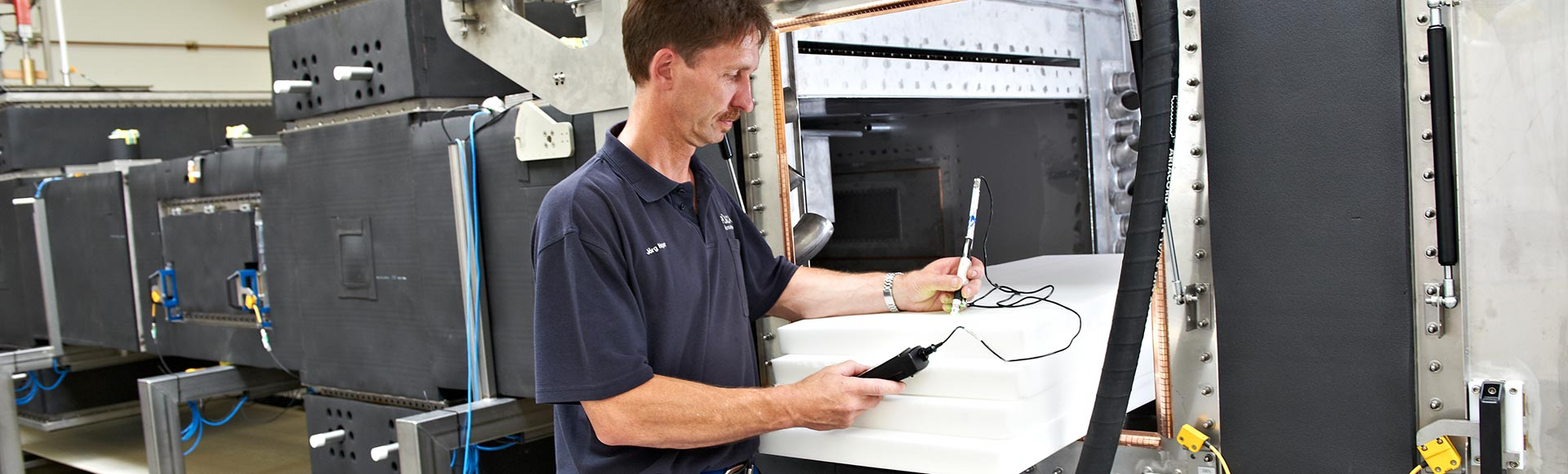•••基本运算

$\mathrm{P\text{'}\text{'}\text{'}}=2\pi f{\epsilon }_{O}{\epsilon }_{r}\mathrm{\text{'}\text{'}}{Ε}^{2}\mathrm{in}W/{m}^{3}$

P´´´ = 体积能量密度，单位W/m3
f = 工作频率，单位赫兹
εo = 自由空间的介电常数 = 8.85 x 10 -12 AS/Vm
ε r´´ = 介电损耗系数，即复介电常数的虚部
Ε = 电场强度，单位V/m（有效值）

• 吸收型，如：水（在25℃时εr’’=12），含水性物质（几乎所有食品），各种塑料制品等。
• 透射型，如：陶瓷石英玻璃（εr’’=0.0023），特氟龙等。
• 反射型，如：金属，石墨等。

$\upsilon =\frac{Ρ\text{'}\text{'}}{{с}_{Ρ}*\rho }=\frac{2800 kW/{m}^{3}}{988 kg/{m}^{3}*4.18kJ/\left(kg * K\right)}$